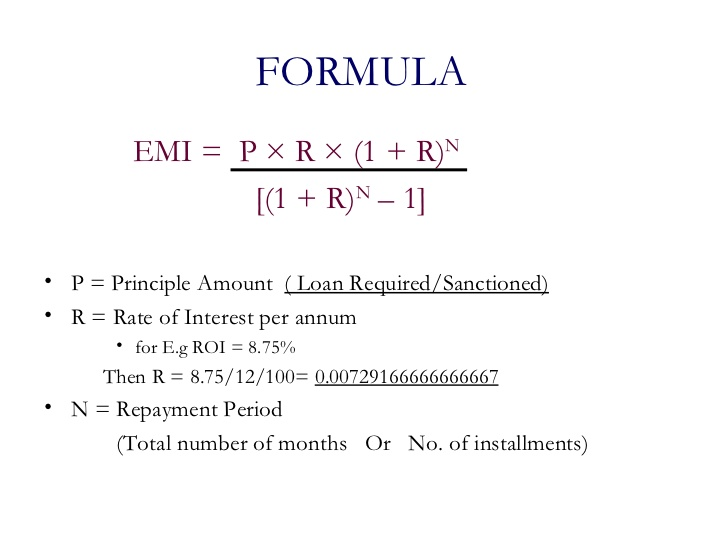# Java Program To Calculate EMI – Monthly & Annum

Java program to calculate EMIs – In this article, we will detail in on all the possible methods to calculate EMIs on a given loan amount in Java programming.

Suitable examples and sample programs have been given in view of each method discussed. The compiler has also been added so that you can execute the programs easily.

The methods used in the article are as follows:

EMI is the abbreviation for Equated Monthly Installments. As the name itself says, it is a monthly breakdown of the amount that you need to repay your loan accordingly.

EMIs play a significant role in our lives where people pay back their dues in a number of installments, instead of actually paying the whole amount off at one go.The formula to calculate EMIs is as follows:

EMI = [P x R x (1+R)^N]/[(1+R)^N-1]

P stands for the principal amount.

R is the rate of interest per month [if the interest rate per annum is 11%, then the rate of interest will be 11/(12 x 100)].

N is the number of monthly instalments.

The rate of interest usually depends on the number of instalments that you are opting for and also on the parent organisation.

The various methods used to calculate EMIs in Java programming are as follows:

1.

Output:
2.
Output:
Output2:
Output3:
3.
Output1:
4.
Output1:
5.
Output:
x

## Java Program to Add Two Matrices – 4 Ways | Programs

Java program to add two matrices – The following Java Code will let you know ...